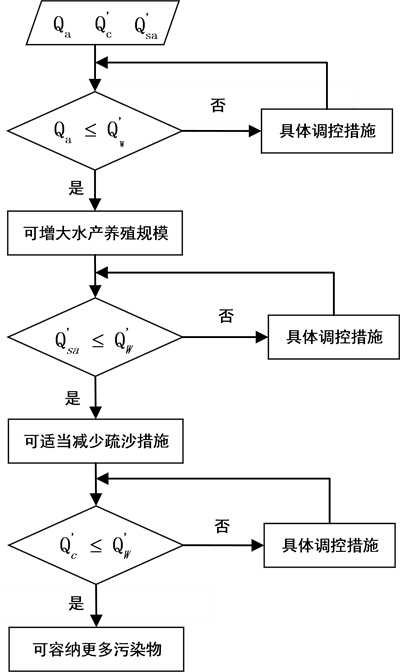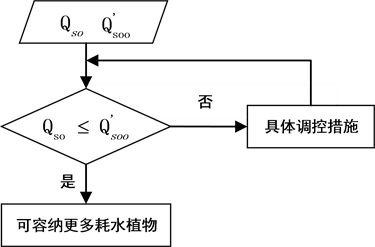﻿ 基于生态需水量的湿地生态系统服务功能研究

# 基于生态需水量的湿地生态系统服务功能研究Research on Ecosystem Services Based on Ecological Water Requirement for Wetland

Abstract: Wetland, an important ecological system, has irreplaceable ecosystem service value. The area of natural wetland decreases rapidly in recent years ; the study on ecosystem service func-tion of wetland becomes a hot topic. Wetland system is a complicated natural system. However, in past several decades, people unilaterally emphasize the function and composition of wetland eco-system or studied as a whole. It becomes a question to study its ecological function with system theory. So, in this paper, the system theory is an integral theoretical basis to study water require-ment of wetland ecosystem. We build a dynamic balance with water requirement of ecology to get the balance equation about wetland system of the ecological water requirement. Then the ideal model is defined as the best function of wetland ecosystem based theory above. The model could avoid the repetition of the different water requirement. In the end, we discuss the application of the model under different management objectives and the best control model. The ideal model could be used for managers to estimate the health of wetland ecosystem, look for the reasons and restore them.

1. 引言

2. 理论基础

3. 研究方法

3.1. 湿地生态需水分项计算方法

3.2. 系统论下的湿地生态需水量模型构建

$±W={P}_{0}+I-R-E$ (1)

$±W={P}_{0}+\left({I}_{s}+{I}_{g}\right)-\left({R}_{s}+{R}_{g}\right)-\left({E}_{s}+{E}_{P}+{E}_{W}\right)$ (2)

$\int \Delta W\text{d}t=\int \left[{P}_{0}+\left({I}_{s}-{R}_{s}\right)+\left({I}_{g}-{R}_{g}\right)-\left({E}_{s}+{E}_{P}+{E}_{W}\right)\right]\text{d}t$ (3)

$\Delta {Q}_{A}=\Delta {Q}_{r}+{Q}_{g}-{Q}_{Eso}-{Q}_{P}-{Q}_{EW}+{Q}_{ra}$ (4)

${{Q}^{\prime }}_{W}+{{Q}^{\prime }}_{SOO}-{Q}_{W}-{Q}_{SOO}=\Delta {Q}_{r}+{Q}_{g}+{Q}_{ra}-{Q}_{ESO}-{Q}_{P}-{Q}_{EW}$ (5)

4. 结论

1) 本文在计算生态需水量时，充分考虑了各种生态功能的生态需水量之间的相关性，避免了当前生态需水量的计算过程中的重复计算问题。

2) 结合水文–生态法和生态功能法，通过地表水系统水量平衡方程进一步推到，探索得到各湿地需水量之间与水量平衡方程中物理量之间的联系，建立湿地各需水量之间的动态平衡方程，实现了湿地各需水量直接的联系。

${{Q}^{\prime }}_{W}+{{Q}^{\prime }}_{SOO}-{Q}_{W}-{Q}_{SOO}=\Delta {Q}_{r}+{Q}_{g}+{Q}_{ra}-{Q}_{ESO}-{Q}_{P}-{Q}_{EW}$

5. 讨论

5.1. 基于地表水蓄水量的湿地理想状态模型的应用

${{Q}^{\prime }}_{c}={Q}_{c}/u$ ; ${{Q}^{\prime }}_{sa}={Q}_{sa}/u$ (6)

${{Q}^{\prime }}_{W}=\Delta {Q}_{r}+{Q}_{g}+{Q}_{ra}+{Q}_{W}+{Q}_{SOO}-{Q}_{ESO}-{Q}_{P}-{Q}_{EW}-{{Q}^{\prime }}_{SOO}$ (7)

5.2. 基于土壤需水量的湿地理想状态模型的应用Figure 1. Control based on surface water storageFigure 2. Control based on soil water requirement

${{Q}^{\prime }}_{SOO}=\Delta {Q}_{r}+{Q}_{g}+{Q}_{ra}+{Q}_{W}+{Q}_{SOO}-{Q}_{ESO}-{Q}_{P}-{Q}_{EW}-{{Q}^{\prime }}_{SOO}-{{Q}^{\prime }}_{W}$ (8)

 Davidson, N.C. (2014) How Much Wetland Has the World Lost? Long-Term and Recent Trends in Global Wetland Area. Marine & Freshwater Research, 65, 936-941.
https://doi.org/10.1071/MF14173

 Niu, Z.G., Zhang, H.Y., Wang, X.W., et al. (2012) Mapping Wetland Changes in China between 1978 and 2008. Chinese Science Bulletin, 57, 2813-2823.
https://doi.org/10.1007/s11434-012-5093-3

 毛德华, 王宗明, 罗玲, 等. 1990-2013年中国东北地区湿地生态系统格局演变遥感监测分析[J]. 自然资源学报, 2016, 31(8): 1253-1263.

 Mao, D.H., Wang, Z.M., Luo, L., et al. (2016) Monitoring the Evolution of Wetland Ecosystem Pattern in Northeast China from 1990 to 2013 Based on Remote Sensing. Journal of Natural Re-sources, 31, 1253-1263.

 国家林业局《湿地公约》履约办公室编译. 湿地公约履约指南[M]. 北京: 中国林业出版社, 2001.

 Bledzki, L.A. Ecosystems and Human Well-Being: Wetlands and Water. Synthesis. http://www.millenniumassessment.org/documents/document.358.aspx.pdf

 Camacho-Valdez, V., Ruiz-Luna, A., Ghermandi, A. and Nunes, P.A.L.D. (2013) Valuation of Ecosystem Services Provided by Coastal Wetlands in Northwest Mexico. Ocean and Coastal Management, 78, 1-11.
https://doi.org/10.1016/j.ocecoaman.2013.02.017

 Armbruster, J.T. (1976) An Infiltration Index Useful in Estimating Low-Flow Characteristics of Drainage Basins. Journal of Research of the US Geological Survey.

 Richter, B., Baumgartner, J., Wigington, R., et al. (1997) How Much Water Does a River Need? Freshwater Biology, 37, 231-249.
https://doi.org/10.1046/j.1365-2427.1997.00153.x

 Smakhtin, V.U. (2001) Low Flow Hydrology: A Review. Journal of Hydrology, 240, 147-186.
https://doi.org/10.1016/S0022-1694(00)00340-1

 Geoffrey, E.P. (1996) Water Allocation to Protect River Ecosystems. Regulated Rivers: Research & Management, 12, 353-365.
https://doi.org/10.1002/(SICI)1099-1646(199607)12:4/5<353::AID-RRR425>3.0.CO;2-6

 杨志峰, 崔保山, 刘静玲. 生态环境需水量理论、方法与实践[M]. 北京: 北京科学出版社, 2003.

 崔保山, 李英华, 杨志峰. 基于管理目标的黄河三角洲湿地生态需水量[J]. 生态学报, 2005, 25(3): 606-614.

 Golbert, J., Gowing, D., Spoor, G., et al. (1996) Quantifying the Hydrological Requirements of Plants as a Tool for the Water Management of Wet Grassland. IEEE.

 (2000) Estimating the Water Requirements for Plants of Floodplain Wetlands: A Guide. Land and Water Resources Research & Development Corporation, Australia.

 董李勤, 章光新, 张昆. 嫩江流域湿地生态需水量分析与预估[J]. 生态学报, 2015, 35(18): 6165-6172.

 奚歌, 刘绍民, 贾立. 黄河三角洲湿地蒸散量与典型植被的生态需水量[J]. 生态学报, 2008, 28(11): 5356-5369.

 赵东升, 吴正方, 商丽娜. 洪河保护区湿地生态需水量研究[J]. 湿地科学, 2004, 2(2): 133-138.

 李加林, 赵寒冰, 刘闯, 等. 辽河三角洲湿地生态环境需水量变化研究[J]. 水土保持学报, 2006, 20(2): 129-134.

 白元, 徐海量, 张青青, 等. 基于地下水恢复的塔里木河下游生态需水量估算[J]. 生态学报, 2015, 35(3): 630-640.

 孙栋元, 杨俊, 胡想全, 等. 基于生态保护目标的疏勒河中游绿洲生态环境需水研究[J]. 生态学报, 2017, 37(3): 1008-1020.

 张长春, 王光谦, 魏加华. 基于遥感方法的黄河三角洲生态需水量研究[J]. 水土保持学报, 2005, 19(1): 149-152.

 周林飞, 许士国, 李青山, 等. 扎龙湿地生态环境需水量安全阈值的研究[J]. 水利学报, 2007, 38(7): 845-851.

 陈敏建, 王立群, 丰华丽, 等. 湿地生态水文结构理论与分析[J]. 生态学报, 2008, 28(6): 2887-2893.

 唐蕴, 王浩, 严登华. 向海自然保护区湿地生态需水研究[J]. 资源科学, 2005, 27(5): 101-106.

 李九一, 李丽娟, 姜德娟, 杨俊伟. 沼泽湿地生态储水量及生态需水量计算方法探讨[J]. 地理学报, 2006(3): 289-296.

 衷平, 杨志峰, 崔保山, 等. 白洋淀湿地生态环境需水量研究[J]. 环境科学学报, 2005, 25(8): 119-126.

 杨柳, 马克明, 白雪, 等. 洪河国家级自然保护区最小生态需水量与补水分析[J]. 生态学报, 2008, 28(9): 4501-4507.

 王效科, 赵同谦, 欧阳志云, 等. 乌梁素海保护的生态需水量评估[J]. 生态学报, 2004, 24(10): 2124-2129.

 崔保山, 杨志峰. 湿地生态环境需水量研究[J]. 环境科学学报, 2002, 2: 219-224.

Top